# SAT Math Multiple Choice Question 787: Answer and Explanation

### Test Information

Question: 787

7. A line in the xy-plane that passes through the coordinate points (3, -6) and (–7, –4) will never intersecta line that is represented by which of the following equations?

• A.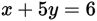• B.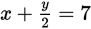• C.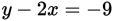• D.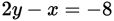Explanation:

A

Difficulty: Medium

Category: Heart of Algebra / Linear Equations

Strategic Advice: Two lines that never intersect are parallel lines, which means they have the same slope.

Getting to the Answer: Start by finding the slope of the line between the two given points: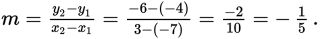Now, find the equation that has a slope of by rewriting the equations in slope-intercept form. Start with (A):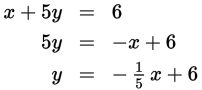The slope of the line is -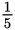, and there can only be one correct answer, so there is no need to check the other choices-(A) is correct.